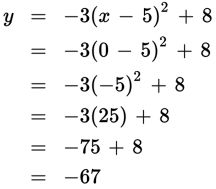# SAT Math Multiple Choice Question 538: Answer and Explanation

### Test Information

Question: 538

13. Given the equation y = -3(x – 5)2 + 8, which of the following statements is not true?

• A. The y-intercept is (0, 8).
• B. The axis of symmetry is x = 5.
• C. The vertex is (5, 8).
• D. The parabola opens downward.

Explanation:

A

Difficulty: Hard